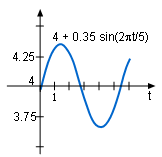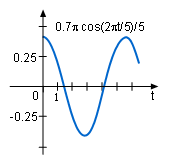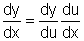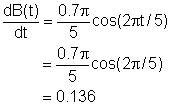Ch 2. Derivatives I Multimedia Engineering Math DerivativeFormulas TrigonometricFunctions Differentiability ChainRule ImplicitDifferentiation
 Chapter 1. Limits 2. Derivatives I 3. Derivatives II 4. Mean Value 5. Curve Sketching 6. Integrals 7. Inverse Functions 8. Integration Tech. 9. Integrate App. 10. Parametric Eqs. 11. Polar Coord. 12. Series Appendix Basic Math Units Search eBooks Dynamics Fluids Math Mechanics Statics Thermodynamics Author(s): Hengzhong Wen Chean Chin Ngo Meirong Huang Kurt Gramoll ©Kurt GramollMATHEMATICS - CASE STUDY SOLUTIONThe brightness of Delta CepheiB(t) = 4 + 0.35 sin(2πt/5)dB(t)/dt = 0.7 cos(2πt/5)/5 The brightness of Delta Cephei increases and decreases according to its brightness function B(t) = 4 + 0.35sin(2πt/b). The brightness of the star b is 5 days. How fast does its brightness changes at time equals one day? Substitute the average brightness of the star 5 days into the brightness function,    B(t) = 4 + 0.35 sin(2πt/5) Let f(t) = 2πt/5, then    B(t) = 4 + 0.35 sin(f(t)) Using the to Chain Rule,the rate of changes for the brightness of Delta Cephei isAfter a day the brightness is